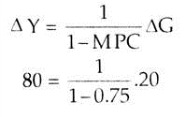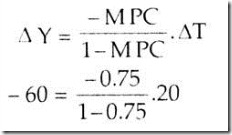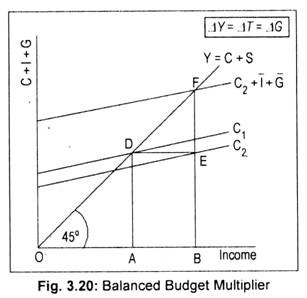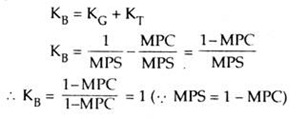# Balanced Budget Multiplier (With Diagram)

We have already considered the indepen­dent effects of government spending and taxes on national income.

Now we will consider the combined effects of government spending and taxes on national income in the light of balanced budget.

Balanced budget means change in government expenditure is exactly matched by a change in taxes. If government expenditure and tax receipts increase by the same amount, will national income or output increase or remain the same?

Classical economists believed that a balanced budget is neutral in the sense that the levels of output or income remain unchanged. However, Keynes and his followers argued that, in reality, its effect on income will not be zero or neutral. In other words, we can find out the expansionary effect on national income of a balanced budget.

The expansionary effect of a balanced budget is called the balanced budget multiplier (henceforth BBM) or unit multiplier. Here an increase in government spending matched by an increase in taxes results in a net increase in income by the same amount. This is the essence of BBM. This may be illustrated here. Let us assume an MPC of 0.75. If government expenditure increases by Rs. 20 crore national income would increase to Rs. 80 crore.

This can be obtained by using the formula for government spending multiplier, KG:Now, an increase in taxes by the same amount (i.e. Rs. 20 crore) would lead to a reduction in aggregate output of Rs. 60 crore.

Applying the formula for tax multiplier, KT, we obtain:This happens because with the increase in taxes of Rs. 20 crore, consumption would decline to Rs. 15 crore and not Rs. 20 crore, since the value of MPC being 0.75. (i.e., 0.75x 20 = Rs. 25 crore). Reduction in consumption by Rs. 15 crore leads to a decline in income by Rs. 60 crore. As a result, the net increase in national income (Rs. 80 – Rs. 60 crore) becomes Rs. 20 crore. Thus, the BBM, defined as the net increase in income (Rs. 20 crore) caused by an increase in government spending (Rs. 20 crore), and increase in taxes (Rs. 20 crore) will have a value of 1. This result is known as the balanced budget theorem or unit multiplier theorem which must have a value of one, no matter whatever the value of MPC.We can explain BBM in terms of the Fig. 3.20 where C1 is the consumption line before the launching of the tax-expenditure programme. C2 is the post-tax consumption line, DE being the tax receipt. If the entire DE amount of tax receipt is spent by the government, the aggregate demand curve would be represented by C2 + I + G, where investment and government expenditure are assumed to be autonomous.

This curve intersects the 45° line at point F. As a result, national income rises from OA to OB. Note that AB = DE = EF.

Since AB represents the increase in income (∆Y), DE is the increase in tax (∆T) and EF is the increase in government expenditure (∆G), we may write:

∆Y = ∆T = ∆G

... ∆Y/ ∆G = ∆Y/ ∆T = 1

The value of BBM (symbolised by KB) is unity. It can also be expressed in the following way:Since KG is positive and KT is negative, the net effect of balanced budget is not neutral. Income changes by an amount equal to a change in government expenditure and tax receipt. So the value of BBM must be 1. Since KT is one less than KG, a balanced budget must have a value of one.

However, in reality, the BBM may have a value less than one. In this model, we assumed the uniform MPC for all taxpayers and beneficiaries of government expenditure. However, if MPC of the taxpayers is different from those of the recipients of government expenditure, the value of BBM would be less than unity, but greater than zero. Thus, the BBM must not have value equal to (one) 1 in a complex society (e.g., in the IS-LM model)